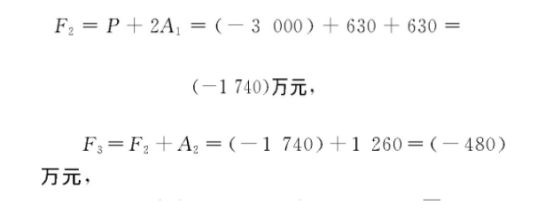西南地区

# 贵州省某地表水热泵能源站项目

1) 现值 (初投资) P=3 000万元, 建设期1a, 运维年限n=20a, 利息为0。

2) 等额年金 (每年的收益) A1=630万元, A2=1 260万元, 计算如下:

A1= (10-3) 元/m2/月×9个月×10万m2=630万元。

A2= (10-3) 元/m2/月×9个月×20万m2=1 260万元。

3) 终值Fi为第i年末的现金总值F4=F3+A2= (-480) +1 260=780万元>0, 投资成本已收回, 且盈利780万元,

F20=P+2A1+18A2=20 940万元 (不考虑残值价值) 。

4) 年投资回报率= (F20-P) /P/21a=28.5%。